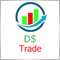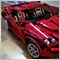# Deal with daily profit %526

Hello everyone,

I have a function that checks my percentage of daily profit. The target profit is 1%, when I took a test by the strategy tester in mt4  with 10 thousand, in the first operation I had a profit of 100.14:

1 2019.01.07 03:50 sell 1 16.69 1.14214 1.14315 1.14208 0.00 10000.00

2 2019.01.07 03:53 t/p 1 16.69 1.14208 1.14315 1.14208 100.14 10100.14

which should exceed 1% of daily profit, but in the Journal it appears:

2019.07.12 00:13:22.365 2019.01.07 04:00:00  ScalpeX EURUSD,M5: Porcentage:0.99147141%

Am I doing something wrong? or has some way of rounding the number if it is for example 0.99% or greater

Thanks

```//+------------------------------------------------------------------+
//|    *ProfitHandler                                                |
//+------------------------------------------------------------------+
bool ProfitHandler()
{
double profit=0;
double porcentageConvert=0;
double profitPorcentageInput =1;
datetime today_midnight=TimeCurrent()-(TimeCurrent()%(PERIOD_D1*60));
for(int x=OrdersHistoryTotal()-1; x>=0; x--)
{
if(OrderSelect(x,SELECT_BY_POS,MODE_HISTORY) && OrderCloseTime()>=today_midnight)
profit=profit+OrderProfit()+ OrderCommission()+OrderSwap();
//Print("Profit:" + DoubleToStr(profit));
}
porcentageConvert=(profit/AccountBalance())*100;
Print("Porcentage:" + DoubleToStr(porcentageConvert)+"%");
if(porcentageConvert>=profitPorcentageInput) return true;

return false;
}```754

Davi Silva:

I have a function that checks my percentage of daily profit. The target profit is 1%, when I took a test by the strategy tester in mt4  with 10 thousand, in the first operation I had a profit of 100.14:

1 2019.01.07 03:50 sell 1 16.69 1.14214 1.14315 1.14208 0.00 10000.00

2 2019.01.07 03:53 t/p 1 16.69 1.14208 1.14315 1.14208 100.14 10100.14

which should exceed 1% of daily profit, but in the Journal it appears:

2019.07.12 00:13:22.365 2019.01.07 04:00:00  ScalpeX EURUSD,M5: Porcentage:0.99147141%

Am I doing something wrong? or has some way of rounding the number if it is for example 0.99% or greater

Did you try without adding commission and swap?526

Seng Joo Thio:

Did you try without adding commission and swap?

Thanks for the suggestion, but it did not work. My solution was to put the variable into static:

```bool ProfitHandler()
{
double profit=0;
datetime today_midnight=TimeCurrent()-(TimeCurrent()%(PERIOD_D1*60));
static double porcentageConvert=(profitPorcentage/100)*AccountBalance();

NormalizeDouble(porcentageConvert,2);
for(int x=OrdersHistoryTotal()-1; x>=0; x--)
{
if(OrderSelect(x,SELECT_BY_POS,MODE_HISTORY) && OrderCloseTime()>=today_midnight)
profit+=OrderProfit()+OrderCommission()+OrderSwap();
//Print("Profit:" + DoubleToStr(profit));
}
Print("Profit:"+DoubleToStr(profit));
Print("porcentageConvert:"+DoubleToStr(porcentageConvert)+"dollars");
if(porcentageConvert<=profit) return true;

return false;
}```